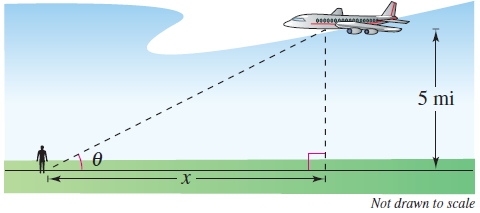×
Get Full Access to Calculus: Early Transcendental Functions - 6 Edition - Chapter 3.6 - Problem 73
Get Full Access to Calculus: Early Transcendental Functions - 6 Edition - Chapter 3.6 - Problem 73

×

# Answer: Angular Rate of Change An airplane flies at an altitude of 5 miles toward aISBN: 9781285774770 141

## Solution for problem 73 Chapter 3.6

Calculus: Early Transcendental Functions | 6th Edition

• Textbook Solutions
• 2901 Step-by-step solutions solved by professors and subject experts
• Get 24/7 help from StudySoup virtual teaching assistantsCalculus: Early Transcendental Functions | 6th Edition

4 5 1 417 Reviews
28
5
Problem 73

Angular Rate of Change An airplane flies at an altitude of 5 miles toward a point directly over an observer. Consider $$\theta$$ and x as shown in the figure.(a) Write $$\theta$$ as a function of x.

(b) The speed of the plane is 400 miles per hour. Find $$d \theta / d t$$ when x = 10 miles and x = 3 miles.

Text Transcription:

theta

dtheta/dt

Step-by-Step Solution:

Step 1 of 5) Symmetry tells us that the integrals of xyz over sides B and C are also 1 > 4. Hence,

Step 2 of 2

##### ISBN: 9781285774770

Since the solution to 73 from 3.6 chapter was answered, more than 211 students have viewed the full step-by-step answer. Calculus: Early Transcendental Functions was written by and is associated to the ISBN: 9781285774770. The answer to “?Angular Rate of Change An airplane flies at an altitude of 5 miles toward a point directly over an observer. Consider $$\theta$$ and x as shown in the figure. (a) Write $$\theta$$ as a function of x.(b) The speed of the plane is 400 miles per hour. Find $$d \theta / d t$$ when x = 10 miles and x = 3 miles.Text Transcription:thetadtheta/dt” is broken down into a number of easy to follow steps, and 64 words. This textbook survival guide was created for the textbook: Calculus: Early Transcendental Functions, edition: 6. This full solution covers the following key subjects: . This expansive textbook survival guide covers 134 chapters, and 10738 solutions. The full step-by-step solution to problem: 73 from chapter: 3.6 was answered by , our top Calculus solution expert on 11/14/17, 10:53PM.

Unlock Textbook Solution